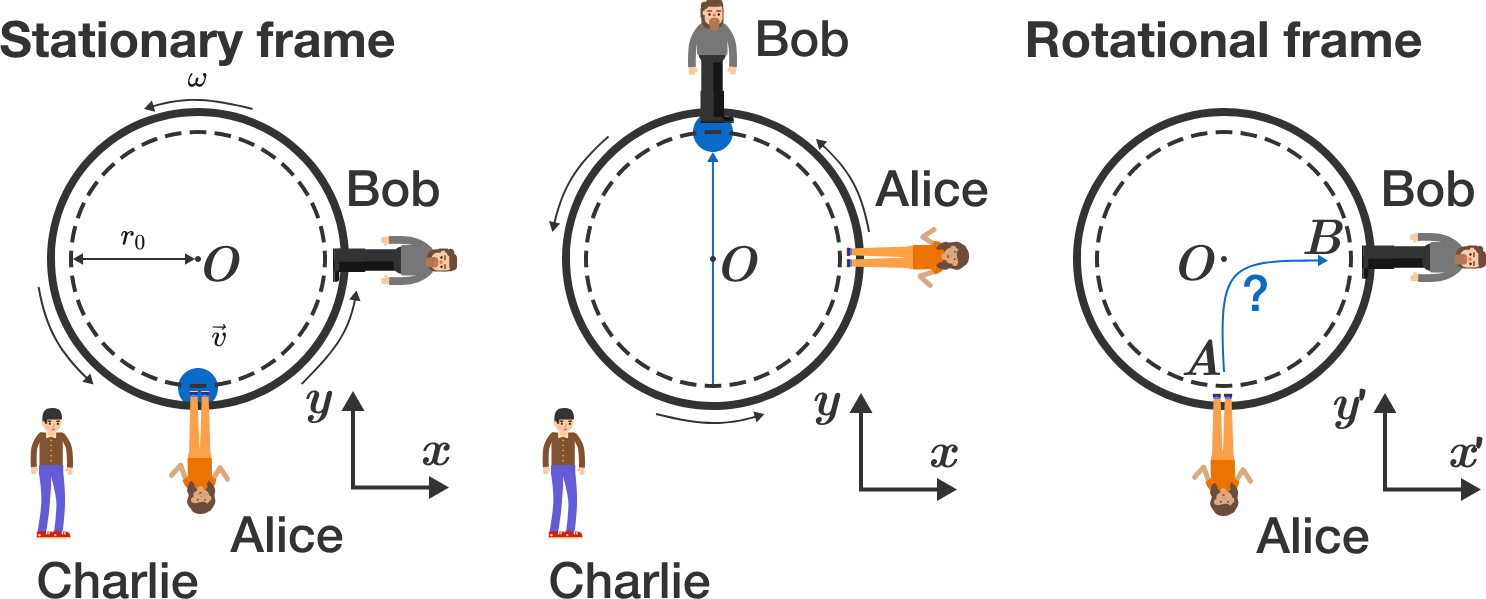# Playground physics

Alice and Bob are having fun throwing a ball to each other on a merry-go-round. Charlie looks at the game from outside of the merry-go-round. From his perspective, the ball thrown by Alice flies straight along the $y$-axis at a constant velocity of $\vec v = v_0 \vec e_y$ to Bob. Bob can catch this ball after the flight time $t_0 = T/4,$ because the merry-go-round has completed a quarter turn in the meantime.But how does Alice observe (rotating reference system)? What average speed (average of absolute value of velocity vector) $\overline{v'} = \frac{1}{t_0} \int_0^{t_0} |\vec v\,'| dt$ does the ball have from Alice's perspective?

Give the answer in units of $v_0$ and with an accuracy of 3 decimal places.


Hints:

• The merry-go-round rotates with constant frequency $\omega = 2 \pi/T$, so that Alice $($point $r_A)$ and Bob $($point $r_B)$ move on circular paths in the stationary reference system. In the rotating reference system, both points $r_A^\prime$ and $r_B^\prime$ are stationary.
• Search for a $2\times 2$ matrix $\mathbf{D}$ such that the transformation reads $\vec{r}^\prime = \mathbf{D} \cdot \vec{r}$. For the calculation of the average velocity, you may use the integral: $\int \sqrt{1 + x^2} d x = \frac{1}{2} \left( x \sqrt{1 + x^2} + \text{arcsinh}(x) \right).$

Bonus question: Which (fictitious) forces act on the ball in the rotating reference system? How can we explain the path of the ball?

×